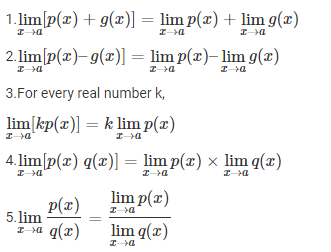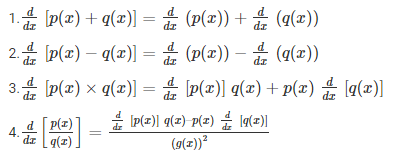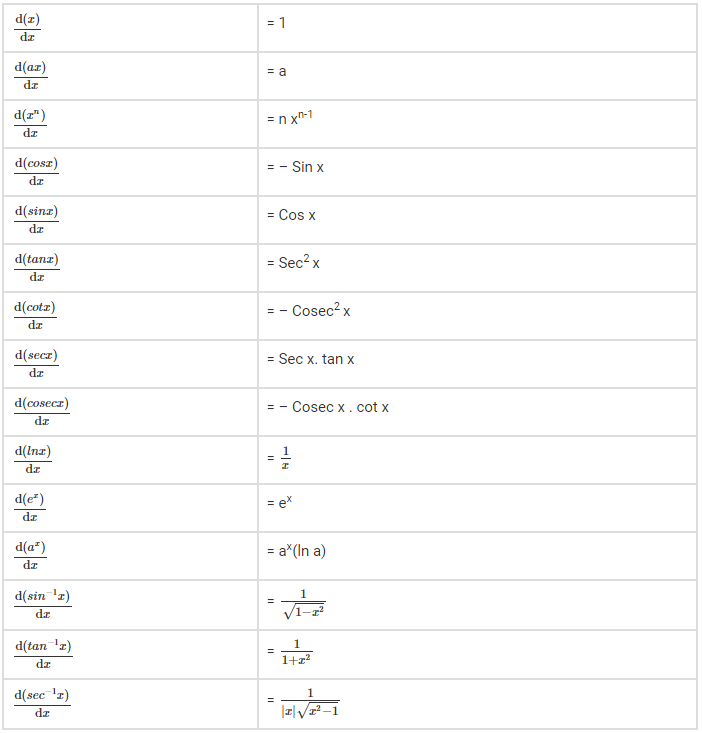# Limits and Derivatives Class 11

Limits and derivatives class 11 covers topics such as intuitive ideas of derivatives, limits, limits of trigonometry functions and derivatives. Limits and derivatives have the scope, not only in Maths but also they are highly used in Physics to derive some particular derivations. We will discuss here Class 11 limits and derivatives syllabus with properties and formulas.

In limits and derivatives topic of class 11, we will cover introduction, definition, formulas, properties of limits and derivatives along with some example questions for both the topics. Let us learn and understand all the concepts in limits and derivatives.

## Limits and Derivatives Class 11 Concepts

### Limits Definition

A limit of a function f(x) is defined as a value, where the function reaches as the limit reaches some value. Limits are used to define integration, integral calculus and continuity of the function.

If f(y) is a function, then the limit of the function can be represented as;

limy→c

This is the general expression of limit, where c is any constant value. But there are some important properties of limits which we will discuss here.

### Properties of limits of a given function

A. Let ‘p’ and ‘q’ be any two functions and ‘a’ be any constant value in such a way that, there exists, limx→a p(x) and limx→a q(x). Now, check below the properties of limits.B. For any positive integers m,

limx→a p(x)(xm-am )/(x-a)= nam-1

C. Limits of trigonometric functions:

If p and q are real-valued function with the same domain, such that, p(x) ≤ q(x) for all the values of x. For a value b, if both limx→a p(x) and limx→a q(x) exists then,

limx→a p(x) ≤ limx→a q(x)

Example:Let f(x) = x2 – 4. Compute $$\lim_{ x \rightarrow 2} f(x)$$.

Solution: $$\lim_{ x \rightarrow 2} f(x)$$ = $$\lim_{ x \rightarrow 2} x^2 – 4$$

= 22 – 4 = 4 – 4 = 0

### Derivatives Definition

A derivative is defined as the rate of change of a function or quantity with respect to others. The formula for derivative can be represented in the form of;

$$\lim_{ a \rightarrow 0} \frac{f(x+a)- f(x)}{a}$$

The derivative of a function f(x) is denoted as f’(x). Now, let us see the properties of derivatives.

### Properties of derivatives for given functions:

Let for functions p(x) and q(x), the properties of derivatives are;Example: Calculate d/dx(x4+1)

Solution: We know,

d/dx(xn)= n xn-1 and derivative of a constant value is 0.

Therefore,

d/dx(x4+1)= 4x3

### General Derivative Formulas## Limits and Derivatives of Class 11 Important Questions and Solutions

1. Question: Evaluate limx→2[(x2-4)/(x-2)].

Solution: limx→2[(x2-4)/(x-2)] = limx→2[(x+2)(x-2)/(x-2)]

Cancel the term x-2 from numerator and denominator. Now we get,

limx→2 x+2 = 2+2 = 4

2. Question: Solve limx→2 (sin 2x/x)

Solution: Given, limx→2 (sin 2x/x)

We can write it as;

limx→2 (sin 2x/2x) × 2

Since, limx→2 (sin x/x)= 1

Therefore, limx→2 (sin 2x/2x) × 2 = 1 × 2 = 2

Get more important questions class 11 Maths chapter 13 limit and derivatives here with us and practice yourself.